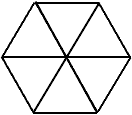hello! I want to know about hexagons. What is the area and the perimeter of an hexagon? Hi, I assume that you are asking about a regular hexagon. If each side is of length a then the perimeter is 6a.To find the area divide the hexagon into 6 equilateral triangles as in the diagram. The area of the hexagon is then six times the area of one of the triangles. The area of an equilateral triangle is explained in Area of a triangle, the answer to a previous question. Cheers, Penny Go to Math Central